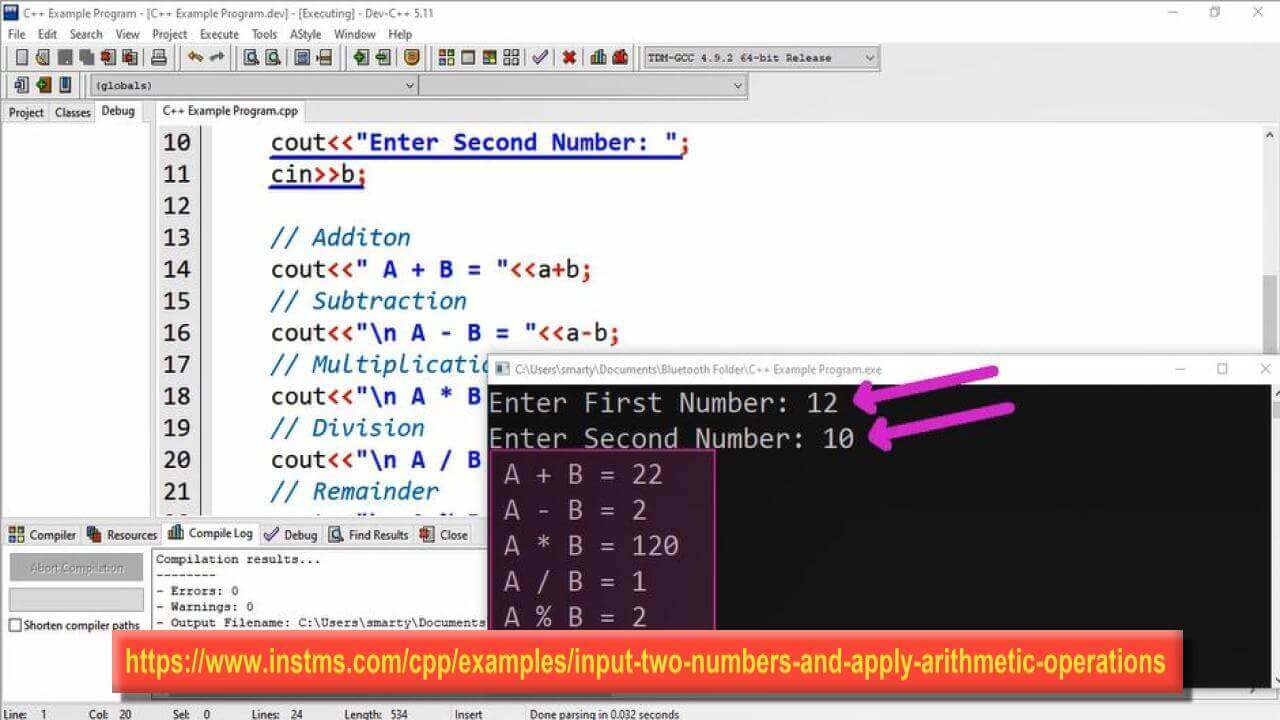# Input Two Numbers And Apply Arithmetic Operations in CPP

## Source Code

#include <iostream>

using namespace std;

int main(int argc, char** argv) {
// Declaration of Two Variables
int a,b;
// Getting Input in Variables
cout<<"Enter First Number: ";
cin>>a;
cout<<"Enter Second Number: ";
cin>>b;

cout<<" A + B = "<<a+b;
// Subtraction
cout<<"\n A - B = "<<a-b;
// Multiplication
cout<<"\n A * B = "<<a*b;
// Division
cout<<"\n A / B = "<<a/b;
// Remainder
cout<<"\n A % B = "<<a%b;
return 0;
}

## Output## Working

This program perform five basic arithmetic operation on input. These operations include

### 5. Remainder

First two variables a and b of type int are declared.Then take input in these variables using cin statement. cout statement is used to display message about input to be taken. These two inputs can also be taken using single cin statement as follow.

cout<<"Enter Two Values : ";

cin>>a>>b;

After getting input all operations are performed in cout statement.